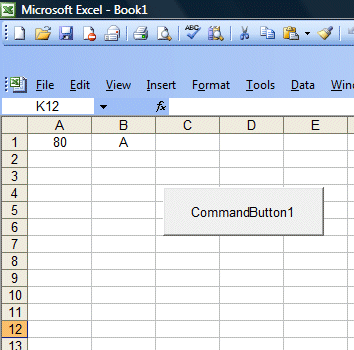# Lesson 13: Using Select Case

The Select Case control structure also involves decisions making but it is slightly different from the If...Then...ElseIf control structure . The If ...Then...ElseIf statement control structure evaluates only one expression but each ElseIf statement computes different values for the expression. On the other hand, the Select Case control structure evaluates one expression for multiple values. Select Case is preferred when there exist multiple conditions as using If...Then...ElseIf statements will become too messy.

The syntax of the Select Case...End Select structure is as follow

Select Case   variable

Case value 1

Statement

Case value 2

Statement

Case value 3

Statement

Case value 4

Statement

.

.

.

.

Case Else

End Select

In the following example, we shall show you how to process the grades of students according to the marks given.

Private Sub CommandButton1_Click()

Dim mark As Single
Dim grade As String
mark = Cells(1, 1).Value
'To set the alignment to center
Range("A1:B1").Select
With Selection
.HorizontalAlignment = xlCenter
End With

Select Case mark
Case 0 To 20
Cells(1, 2) = grade
Case 20 To 29
Cells(1, 2) = grade
Case 30 To 39
Cells(1, 2) = grade
Case 40 To 59
Cells(1, 2) = grade
Case 60 To 79
Cells(1, 2) = grade
Case 80 To 100
Cells(1, 2) = grade
Case Else
Cells(1, 2) = grade
End Select

End Sub

¡¡

You should also include the error case where the values entered are out of the range or invalid. For example, if the examination mark is from 0 to 100, then any value out of this range is invalid. In this program, we use case else to handle the error entries.

¡¡

The Figure below shows the output of this example.